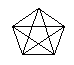Name: ___________________Date:___________________

 Email us to get an instant 20% discount on highly effective K-12 Math & English kwizNET Programs!

### MEAP Preparation - Grade 4 Mathematics3.10 Diagonals

 A diagonal is a line segment that is not a side and that joins two vertices of a polygon. Directions: Answer the following. Also draw a triangle, square and rectangle and show its diagonals.Name: ___________________Date:___________________

### MEAP Preparation - Grade 4 Mathematics3.10 Diagonals

 Q 1: If the diameter of a circle is 28 meters, what is the radius?28 meters14 meters2 meters85 meters Q 2: The diagonals of a rhombus areparallel to each otherperpendicular to each other Q 3: A diagonal is a line segment that is not a side and that joins two vertices of a polygon.truefalse Q 4: A quadrilateral has ___ diagonals.342 Q 5: A pentagon has ______diagonals.6754 Q 6: A hexagon has ______diagonals.698 Question 7: This question is available to subscribers only! Question 8: This question is available to subscribers only!